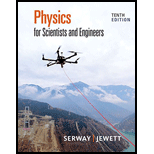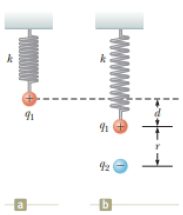Chapter 22, Problem 32AP### Physics for Scientists and Enginee...

10th Edition
Raymond A. Serway + 1 other
ISBN: 9781337553278

#### Solutions

Chapter
Section### Physics for Scientists and Enginee...

10th Edition
Raymond A. Serway + 1 other
ISBN: 9781337553278
Textbook Problem

# A small sphere of charge q1 = 0.800 μC hangs from the end of a spring as in Figure P22.32a. When another small sphere of charge q2 = −0.600 μC is held beneath the first sphere as in Figure P22.32b, the spring stretches by d = 3.50 cm from its original length and reaches a new equilibrium position with a separation between the charges of r = 5.00 cm. What is the force constant of the spring?Figure P22.32To determine

The force constant of the spring.

Explanation

Charge on a small sphere is 0.800 μC, charge on the sphere beneath the first sphere 0.600 μC, displacement of spring from its original position 3.50 cm and separation between the two charges 5.00cm.

Write the expression for the expression for the spring force according to Hooke’s law

Fs=kd                                                       (1)

Here, Fs is the spring force, k is the spring constant and d is the displacement of the spring

Write the expression for the electric force according to Coulomb’s law

F=keq1q2r2

Here, F is the force due to electric field, ke is the Coulomb’s constant, q1 is the charge on the first particle, q2 is the charge on the second particle and r is the separation between the two charges.

Substituting 8.99×109Nm2/C2 for ke, 0.8 μC for q1, 0.6 μC for q2 and 5 cm for r to get F in the above equation,

F=(8

### Still sussing out bartleby?

Check out a sample textbook solution.

See a sample solution

#### The Solution to Your Study Problems

Bartleby provides explanations to thousands of textbook problems written by our experts, many with advanced degrees!

Get Started

## Additional Science Solutions

#### Find more solutions based on key concepts